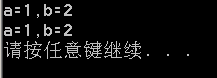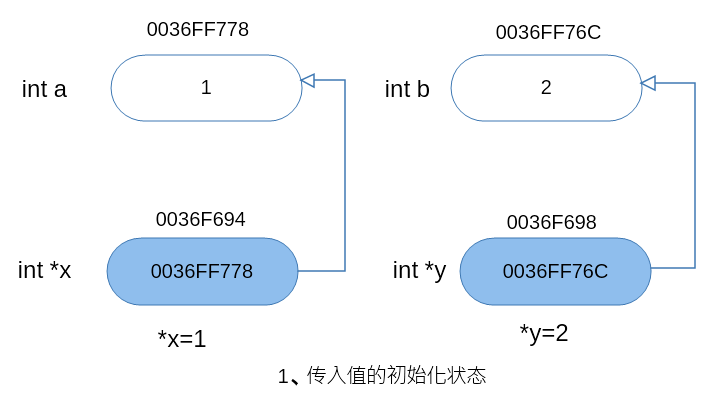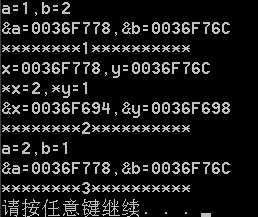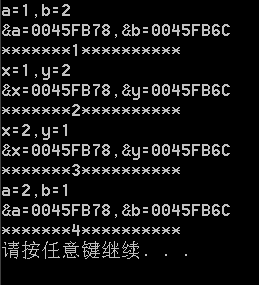# C++函数调用之——值传递、指针传递、引用传递

### 1、简介

1、值传递：形参时实参的拷贝，改变函数形参并不影响函数外部的实参，这是最常用的一种传递方式，也是最简单的一种传递方式。只需要传递参数，返回值是return考虑的；使用值传递这种方式，调用函数不对实参进行操作，也就是说，即使形参的值发生改变，实参的值也完全不受影响。

2、指针传递：指针传递其实是值传递的一种，它传递的是地址。值传递过程中，被调函数的形参作为被调函数的局部变量来处理，即在函数的栈中有开辟了内存空间来存放主调函数放进来实参的值，从而成为一个副本。因为指针传递的是外部参数的地址，当调用函数的形参发生改变时，自然外部实参也发生改变。

3、引用传递：被调函数的形参虽然也作为局部变量在栈中开辟了内存空间，但在栈中放的是由主调函数放进来的实参变量的地址。被调函数对形参的任何操作都被间接寻址，即通过栈中的存放的地址访问主调函数中的中的实参变量（相当于一个人有两个名字），因此形参在任意改动都直接影响到实参。

### 2、例程

##### 1、值传递
#include <iostream>
using namespace std;
void swap(int,int);
int main()
{
int a=1,b=2;
cout<<"a="<<a<<",b="<<b<<endl;
swap(a,b);
cout<<"a="<<a<<",b="<<b<<endl;
return 0;
}
void swap(int x,int y)
{
int p=x;
x=y;
y=p;
}##### 2、指针传递
#include <iostream>
using namespace std;
void swap(int *x,int *y);
int main()
{
int a=1,b=2;
cout<<"a="<<a<<",b="<<b<<endl;
cout<<"&a="<<&a<<",&b="<<&b<<endl;
cout<<"********1**********"<<endl;
swap(&a,&b);
cout<<"a="<<a<<",b="<<b<<endl;
cout<<"&a="<<&a<<",&b="<<&b<<endl;
cout<<"********3**********"<<endl;
return 0;
}
void swap(int *x,int *y)
{
int p=*x;   //int p; p=*x;
*x=*y;
*y=p;
cout<<"x="<<x<<",y="<<y<<endl;
cout<<"*x="<<*x<<",*y="<<*y<<endl;
cout<<"&x="<<&x<<",&y="<<&y<<endl;
cout<<"********2**********"<<endl;
}

int *x=&a;//用于指针传递，x有自己独立的内存地址，存储内容是a的地址，*x是存a的值。##### 3、引用传递
#include <iostream>
using namespace std;
void swap(int &x,int &y);
int main()
{
int a=1,b=2;
cout<<"a="<<a<<",b="<<b<<endl;
cout<<"&a="<<&a<<",&b="<<&b<<endl;
cout << "*******1**********"<<endl;
swap(a,b);
cout<<"a="<<a<<",b="<<b<<endl;
cout<<"&a="<<&a<<",&b="<<&b<<endl;
cout << "*******4**********"<<endl;
return 0;
}
void swap(int &x,int &y)
{
cout<<"x="<<x<<",y="<<y<<endl;
cout<<"&x="<<&x<<",&y="<<&y<<endl;
cout << "*******2**********"<<endl;
int p=x;
x=y;
y=p;
cout<<"x="<<x<<",y="<<y<<endl;
cout<<"&x="<<&x<<",&y="<<&y<<endl;
cout << "*******3**********"<<endl;
}posted @ 2018-08-16 01:35  小猪利琦  阅读(13758)  评论(3编辑  收藏  举报